## General Mechanical

Topics relate to Mechanical Enterprise, Motion, Additive Print and more

•Adisa
Subscriber

Hi everyone,

I did simple analysis of c. beam. I used nonlinear material, PA GF15. I converted engineering stress and strain to true.

In the results I did not get corresponding curve of stress strain.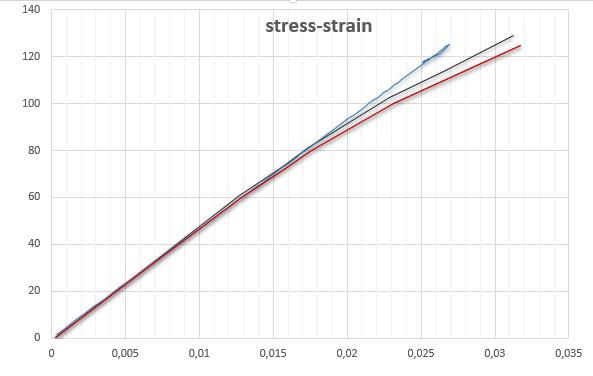red - Eng. stress-strain,

black - True stress-strain,

blue - the result stress-total strain.

I wanted to determine max. Force which should be occur in max. stress (in the above pic. it is about 120MPa.), but the result of stress and strain behave as linear not as nonlinear, which is needed.

I sent the data sheet of material and the archive file of the analysis.

Whether I have determined Young module correct.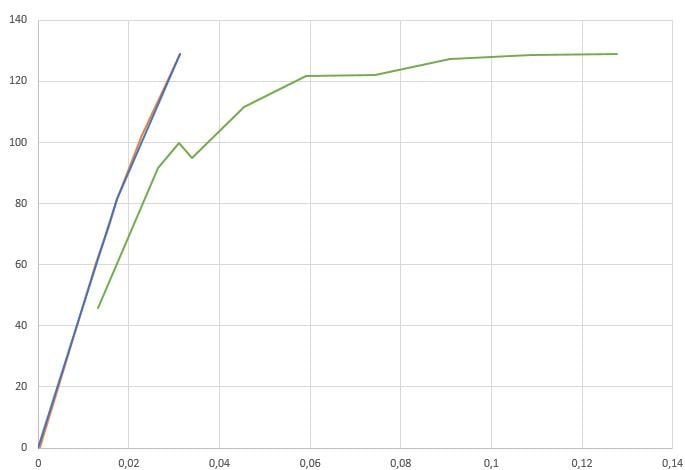In the pic. above I used PA GF15 plastic material for more complex geometry where is used tetrahedron elements but green curve is result of stress strain, but blue is entered as bilinear isotropic hardening. As the pic shows the results do not respond the input data stress and strain of plastic material.

All the best good people!!!

•peteroznewman
Subscriber

MULTILINEAR PLASTICITY
A single element in a pure tensile test is a good way to validate a plasticity material model. A pure tensile stress is developed if three displacement supports, one for each of three orthogonal faces, are provided. Then a force on the end creates a tensile stress. The cross-sectional area of your beam is 75 sq. mm so a force of 10,000 N creates a stress of 132 MPa.

Look at your Multilinear Plasticity Model. It only goes up to a strain of 0.002451 and a stress of 129 MPa which means the solver can't go past that point.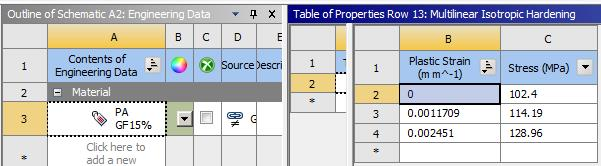Look at the change in slope at 114 MPa, there is almost no change in slope at that point and the change is hardening, not softening, which is what I would expect to see.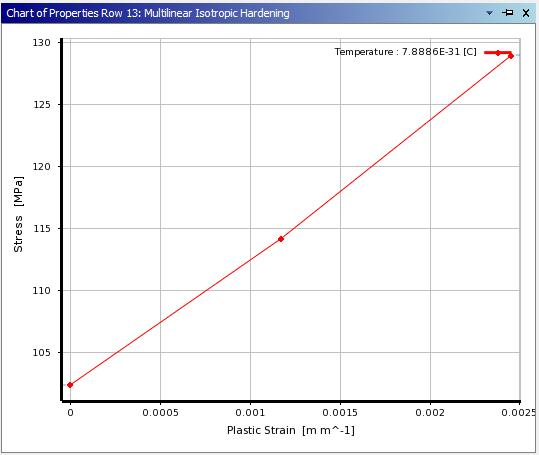When I tried to solve my 4 element model, it stopped with an error at t=0.96 when it got to the end of this table.
Below is a revised table that goes to higher values of stress and strain and there is a significant change in slope at 129 MPa.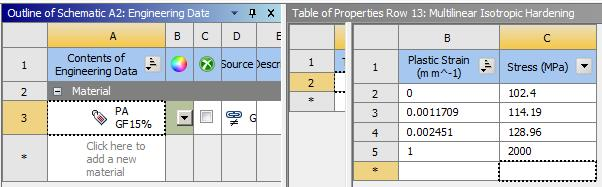Now the solver will get to the load requested. Here is the chart you created of equivalent stress vs total strain. The change in slope at the yield point of 102 MPa is visible, as is the change in slope at 129 MPa at the last point you defined. The last few points are on the new segment I added at the end.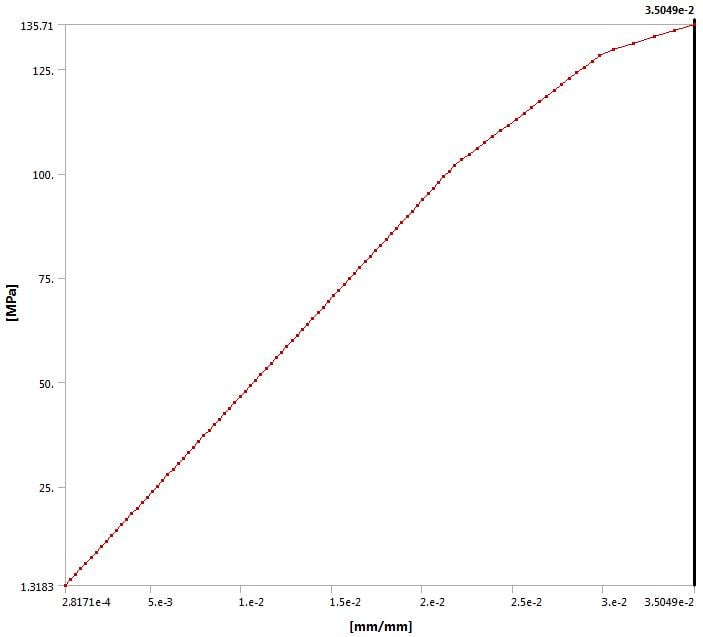VENDOR DATA
The vendor provided two graphs, one labeled dry, one labeled cond. They show very different Stress-strain curves at the same temperature.

You should read this to learn about the difference between dry and conditioned parts. You seem to have taken the data from the dry graph, but that is not representative of parts in use. You should be using the data from the cond. graph on the right.

Kind regards,

Peter

ANSYS 18.2 archive is attached.

•Adisa
Subscriber

Peter, thank you so much.

Please correct me if I am wrong.

As we can see in Hooks diagram-dry max stress is  125 MPa and strain is 3 %, and in the properties of this material those stress - strain are breaking criteria for tem 23?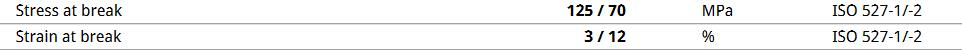I am confused, I have some question. If anyone knows, please tell me.

1.  Whether the force  at  max. stress 125 MPa and strain 3 % is max force that will occur on the  construction for the material of the last post? And this  point (125 MPa - 3 %) means the fracture point, the construction will break? In the example from the last post, the fracture force will be smaller than a force of 10,000 N, because this force creates a stress of 132 MPa and the fracture stress is 125.

We can see the stress after max stress start to decrease and at elongation 40 % will occur fracture of the construction.

In the above link,  the max force is 630 N and occurs at max. stress. I do not know how to analyze after max stress, from max stress to stress at fracture (40% elongation). In ansys I can input decreasing stress after max stress. Whether I can do this analysis in static or i must need another type of analysis.

Maybe for the  fracture criteria I need to use elongation (40%) no stress criteria and assume that stress from max to stress at elongation 40% is constant

The elongation of 40 %, how can I get it, will I use force higher than 630 N, for example 700N (assumption that at force of 700 N will occur the elongation of 40%).  And that force of action (assumed force of 700N) not equal force of reaction (as we can se form the above video,  the breaking force is about of 488 N). And can I say that breaking of the construction will occur at 700 but at 630 N the construction can be used in real life?

In The below link has one more example of tensile test.

If anyone want to help me that verify this analysis in the above link.

3. Can Hooks diagram use for all loads (bending) or it we can use only for tensile.

I am trying to do max. force for panel clips, but an analysis did not converge. I always have problems with non linear contact. I attached the archive file.

Best regards.

•peteroznewman
Subscriber

There is much to explain about materials, but I will save that for a later post. I want to address why your model did not converge. It has nothing to do with your materials and has everything to do with your geometry.

When using contact, any body that is entirely supported by contact must be touching other bodies to create a load path to ground. Your spring clip is not touching any other body. It is just floating free in space. The solver cannot start from this configuration. That is why it failed to converge, there is no load path to ground.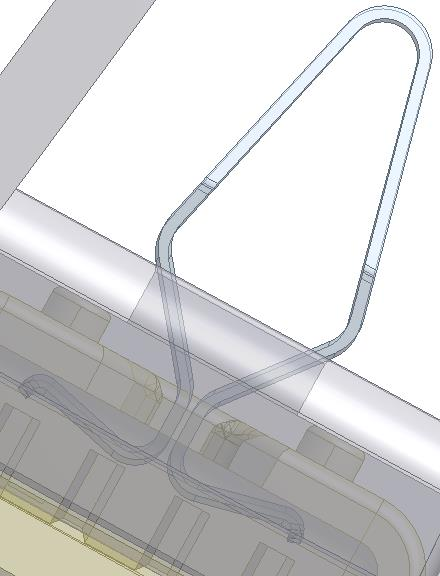Your setup has the large grey part fixed to ground, while the yellow part will be pushed down and pivot at the other end. You can see in the image above that the spring clip is not in contact yet. It is nearly in contact with the grey part, but it has a small clearance. There is a larger clearance to the yellow part.

The corrective action is to move the spring clip down (at a 30 degree angle) a very small amount until it is touching the rounds on the edge of the hole in the grey part. Then you have to move the yellow part down a small amount until it is touching the bends on the top of the spring clip. Once you have the parts in the correct positions where they are all just touching, you will give the solver a chance to iterate to convergence on the first substep.

RMB on Connections branch in the Outline and Insert a Contact Tool.  RMB and Generate Initial Contact Results. This will tell you if you have gaps.  A small penetration is much easier for the solver to resolve than a small gap.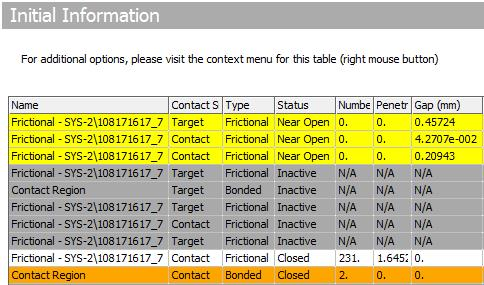Once you make those geometry changes, please post a new archive.

Regards,
Peter

•peteroznewman
Subscriber

1.  Whether the force at max. stress 125 MPa and strain 3 % is max force that will occur on the construction for the material of the last post? And this  point (125 MPa - 3 %) means the fracture point, the construction will break?

The manufacturer's data tells us that if the dry material is under a tensile stress and the strain has reached 3%, that means fracture is predicted. The model results will not show the fracture, they will show the total strain has reached 3%.  If you increase the load, you can simulate 4, 5 and 6% strain. You don't see failure in the model, you predict failure in the part from the simulation results. If the load you applied resulted in 6% strain, then look at the history of strain and look at the time when 3% was reached. Multiply that time by the applied load to determine the ultimate load. In the example you attached above, the load goes up to 60 N, which was not enough to get past 3% total strain.

•peteroznewman
Subscriber

I do not know how to analyze after max stress, from max stress to stress at fracture

I'm really impressed with campusplastics.com. They provide a graph and the data!

This is a table of Engineering Stress and Engineering Strain. The cross-sectional area is not updated during the test so True Stress is under predicted. This must be corrected to create data for Multilinear Plasticity. I wrote about that in this discussion.

Those corrections are only valid until necking occurs, which is when the force (or Engineering Stress) curve reaches the peak and begins a negative slope. In the table above, that is at a strain of 9.1%. The next points cannot be used to create data for Multilinear plasticity table in ANSYS.

Yet the sample continues to be pulled until the Engineering strain reaches 12.5%. The best way to resolve this is by building a model of the tensile test sample that includes the necking behavior. Estimate stress values in the table above 9.1% and run a simulation of the tensile test. This will generate stress-strain results during necking that can be plotted and compared with the real test data. The errors between the simulated and real test data can be used to update the values of stress in the multilinear plasticity table and after a few iterations, a reasonable agreement between the engineering stress-strain profile of the real test and the simulation with the tuned material table will be obtained.

•peteroznewman
Subscriber

The elongation of 40 %, how can I get it, will I use force higher than 630 N, for example 700N

If you build a model with plasticity, you can't apply a force to stretch the part to 40% elongation, because when the force-displacement curve reaches the peak, there are no more static equilibrium points with higher forces, so the solution stops.

You have to apply a displacement BC to the end instead of a force BC. Then you can continue to displace the end out past 40% strain, as long as the element shape does not become too distorted to compute accurate results. Excessive element distortion is a common reason for the solver to stop before the full applied displacement is reached. If you have an applied displacement BC, you insert a Force Reaction plot to get the Force results, which you can plot against the displacement to create the Instron curve.

3. Can Hooks diagram use for all loads (bending) or it we can use only for tensile.

Yes. For ductile material, the von Mises equivalent stress converts a complex 3D state of stress to a single number that is compared directly to the normal stress in a tensile test. The part will start yielding when the equivalent stress exceeds the yield strength that was determined in a tensile test.

You can show your appreciation by clicking Like below the posts that are helpful.

•Adisa
Subscriber

Hi Peter,

Thank you so much. I am learning a lot here in the community, thank you.

The correction geometry from the last post, the solver did not converge.

Has anyone good tutorial for the nonlinear contacts.

I am so confused about non linear contacts, has anybody recommend some link, book etc.

•peteroznewman
Subscriber

I don't understand why your remote displacement is so far away from the part being moved.
In the image below, it is way off to the side and a long distance in -Z, look near the 20.00 (mm) scale.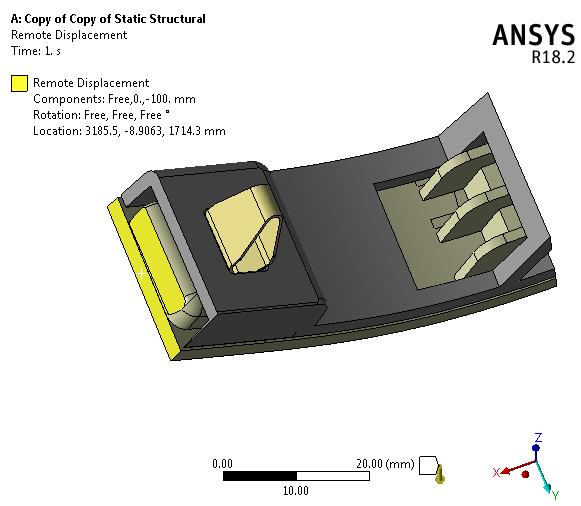I created a new Remote Displacement on the yellow face on the part.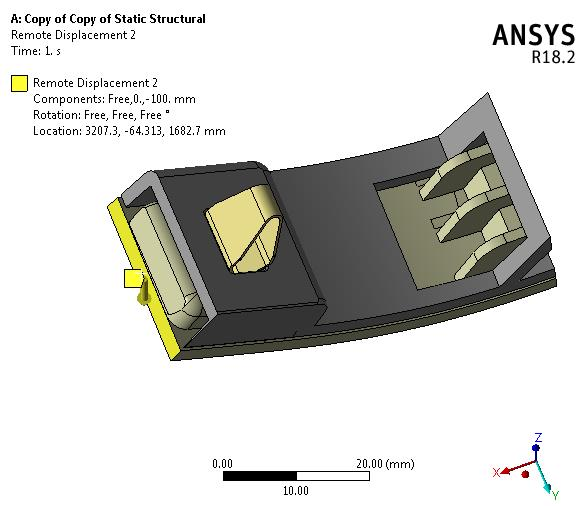I created two Remote Displacements, a temporary one that held a face in all six DOF at 0 and a second Remote Displacement that only held the Z DOF.
I created a Coordinate System aligned as shown below and I am pulling the face only 10 mm in this -Z direction.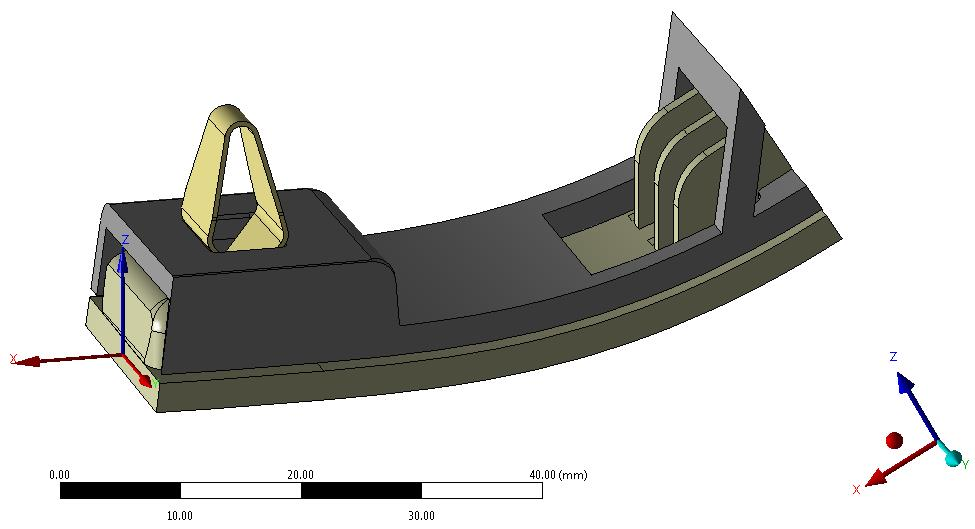You need a better mesh on the spring clip. Below is a good one.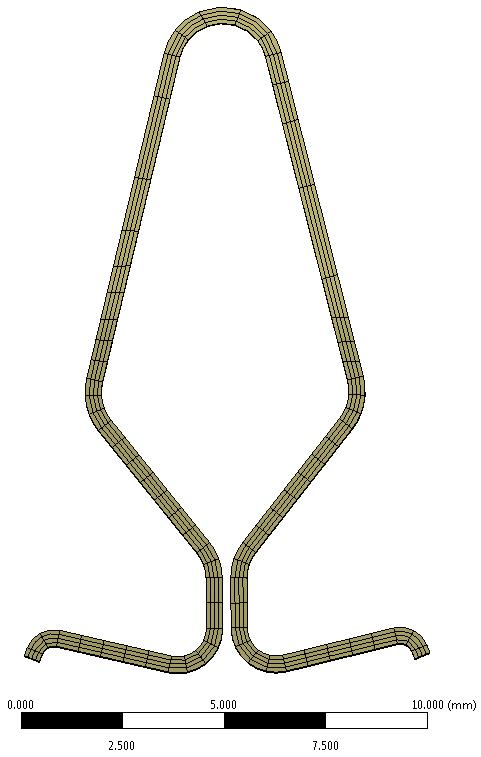That is going to be costly in solution time.

(1) You could replace it with 1/5 as many elements if you take a midplane and use shell elements.

(2) You could dramatically ease the problem of convergence by applying Symmetry through the center of the spring. That immediately cuts the DOF in half and makes it much more likely to solve. What you lose is the ability to have non-symmetric results.

(3) You could also ease convergence issues by concentrating on the spring and using a revolute joint at the hook end.

(4) You could make the two thick parts rigid bodies, and just leave the spring clip flexible.

I have implemented those four suggestions in the model below.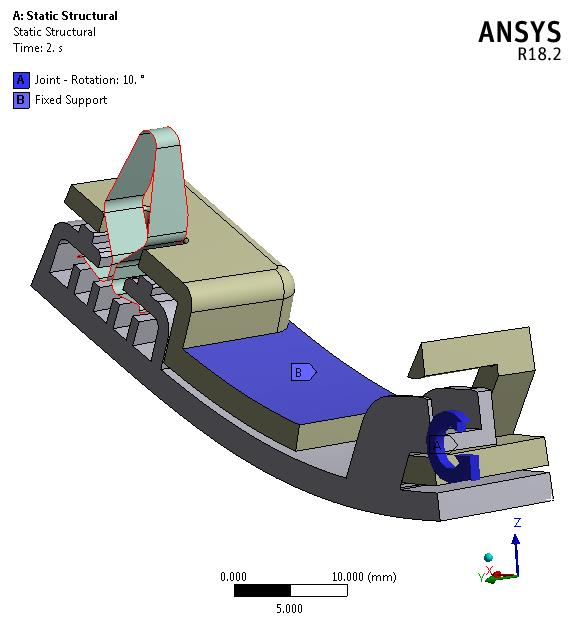I created a 2 step solution.
Step 1 hold revolute at 0 degrees to let the contacts resolve the initial penetration.
Step 2 ramp revolute rotation to 8 degrees in 400 substeps.

With this degree of simplification, the model converges up to the point when the spring clip goes from being pulled to pushing. At that point, there is no static equilibrium for the next increment of displacement because the spring clip wants to jump the gap in the assembly. You can only track that sort of behavior in a Transient Dynamics solver.

Right click on this video and select Loop so you can see it over and over.

https://youtu.be/uNSZjwURMYA

Attached is an ANSYS 18.2 archive.

•Adisa
Subscriber

Peter, Thank you.

You are the best teacher, thank you for the extra effort.

Yes, the problem is solved.

Best regards.